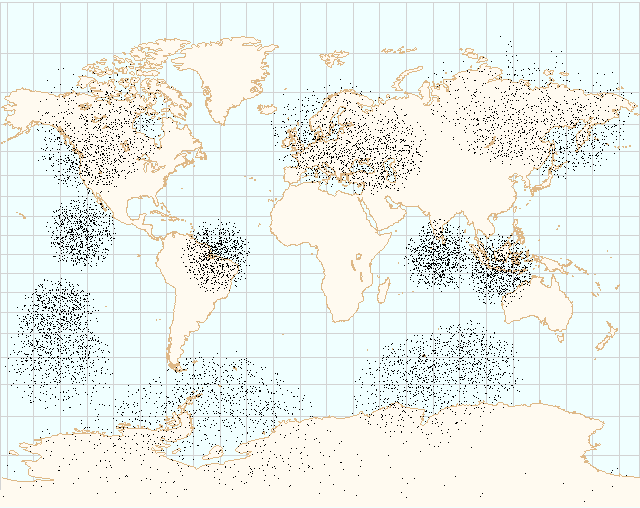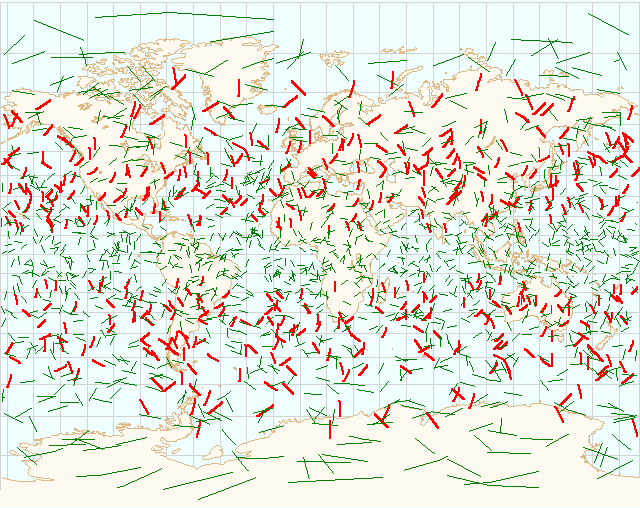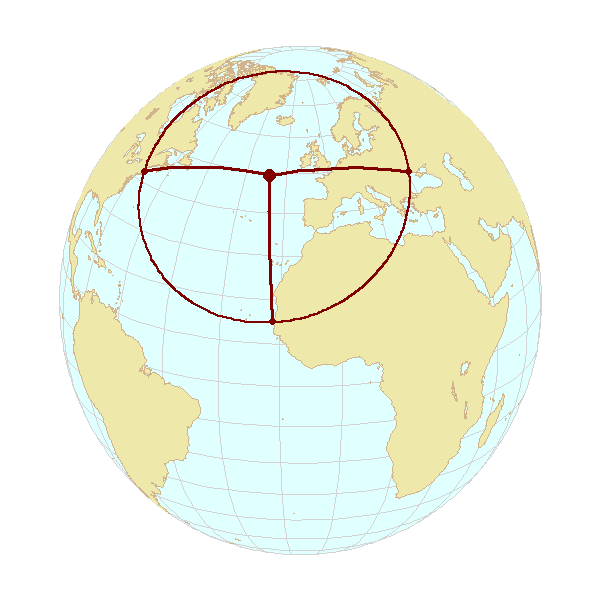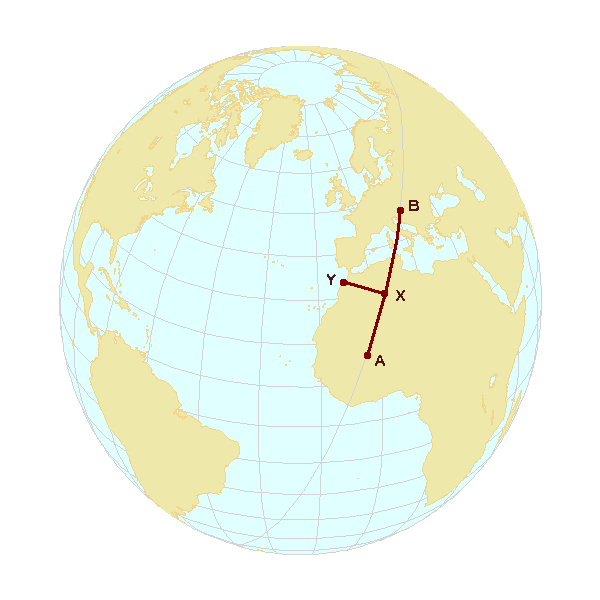Retrun to document top page

### Nemo Library, section 3

Spherical surface geometry productions
```   Functions performing Nemo Library geometry productions on the surface
of a sphere. In this library, the sphere will typically be an
ellipsoid-specific near-conformal unit sphere, "NCS". See the
preamble of the ellipsoid geometry library section for additional
NCS commentary.
```

 nemo_SphereRandomLocation() Generate a random location on unit sphere nemo_SphereRandomLine() Generate random line on unit sphere nemo_RandomLocal() Generate regional random point on unit sphere nemo_SphereCircumcenter() Find spherical circumcenter nemo_SphereTangentialPlane() Principal vectors of tangential plane nemo_SphereChordDirect() Direct problem of geodesy on sphere nemo_SphereChordInverse() Inverse problem of geodesy on sphere nemo_SphereChordCoords() Spherical Abscissa and Ordinate nemo_SphereSegsXsct() Intersection of two great circle segments

nemo_SphereRandomLocation()
```Synopsis:
#include <nemo.h>
nemoPtNcs *nemo_SphereRandomLocation(nemoPtNcs *pRandNcs);
```Test data generation example: 16 clusters of random global locations

```Description:
Generate a random, uniformaly distributed location on a unit sphere.
·
The function starts by generating a random point in a cube tightly
encompassing a sphere with RAND_MAX radius. Points are rejected if outside
the sphere, or if their radius-vector is so small that the normalization
might be numerically problematic; otherwise their radius-vector is
normalized. Assuming original points are generated with uniform density
inside the cube, the resulting points will cover the surface of the
sphere with an "uniformly random" distribution. Using this function as
a "primitive", an application can (see the illustration above) generate
specific patterns of globally distributed points to fit specific
geometry tests or spatial algorithms benchmarking scenarios.
·
The function uses C math library rand() function, see its documentation
for the consequences of seeding it (or absence of it). Hint: an application
should probably call srand(time(0)) before the first call to this function.
Argument:
pRandNCS:
Pointer to nemoPtNcs structure, returned random point or NULL.
If the argument is NULL, an internal static structure memory will
be used for generated location, in which case the function will
Return Value:
Pointer to the nemoPtNcs structure with the generated random location
on the unit sphere. (cf., pRandNCS argument).
nemo_SphereRandomLine()   nemo_RandomLocal()```

nemo_SphereRandomLine()
```Synopsis:
#include <nemo.h>
void nemo_SphereRandomLine(const nemoPtNcs *ptNcs,
double arc,
nemoPtNcs *pRlnNcsA,
nemoPtNcs *pRlnNcsB);
```Approximation of geodesic by NSC arc scaled to ellipsoid.

```Description:
Given a line mid-point, generate a random line of a given arc length
on the unit sphere. Similar to the nemo_SphereRandomLocation(), this
function is used in testing, benchmarking and visual confirmation of
geodetic propositions; as in the map above, which illustrates the dependency
on latitude of the quality of approximation of geodesic using NCS arc
scaled up to the ellipsoid (using NCS to ellipsoid scale factor calculated
by nemo_NcsElrScale() at the line mid-point).
Arguments:
ptNcs:
Pointer to nemoPtNcs structure, mid-pint of the line
(usually generated using nemo_SphereRandomLocation() function;
arc:
double, the length of the line, defined by its spherical arc,
(with π as the maximum).
pRlnNcsA, pRlnNcsB:
Pointers to nemoPtNcs structure, returned start and end point of
the line.
nemo_SphereRandomLocation()   nemo_RandomLocal()   nemo_SphereChordDirect()```

nemo_RandomLocal()
```Synopsis:
#include <nemo.h>
void nemo_RandomLocal(const nemoPtNcs *ptNcs,
double arc,
double parms[NEMO_GNOMONIC_PCNT],
nemoPtNcs *pRandNcs);
Description:
This function is similar to its two sibling, but instead of creating a
random object uniformly distributed over the entire planetary surface, the
object (point) is distributed, with somewhat less uniform distribution,
over a smaller region defined by its centre and unit sphere arc radius.
·
The function internally generates a random point in the gnomonic projection
plane, and "un-maps" the planar point back to sphere. It is therefore quite
magnitude smaller that the planet radius.
.
In addition to its primary purpose, the function provides an example of
purposeful use of spherical gnomonic projection to deal with the spherical
phenomena of limited spatial extent.
Arguments:
ptNcs:
Pointer to nemoPtNcs structure, center of the region of the required
set of uniformally-distributed random spherical points.
arc:
Unit sphere radius of the region.
parms:
An array of doubles, Gnomonic projection parametars. Before the first
invocation of the function in the ptNcs vicinity, the element  of
the array should be set to  NEMO_DOUBLE_UNDEF so that the function code
can initialize the array (by an internal call to nemo_GnomonicInit()).
pRandNcs:
Pointer to nemoPtNcs structure, returned random point or NULL.
If the argument is NULL, an internal static structure memory will
be used for generated location, in which case the function will
Return Value:
Pointer to the nemoPtNcs structure with the generated random location
on the unit sphere (cf., pRandNCS argument), the locus as defined above.
nemo_SphereRandomLocation()   nemo_GnomonicInit()```

nemo_SphereCircumcenter()
```Synopsis:
#include <nemo.h>
int nemo_SphereCircumcenter(const nemoPtNcs *pA,
const nemoPtNcs *pB,
const nemoPtNcs *pC,
nemoPtNcs *pCntr);
```Circumcenter of Odessa, Boston and Dakar

```Description:
Given are three points on the sphere, find the point that is
equidistant from all three given points.
·
Since on the sphere there are always two such points - "near" and
"far", the order of three given points is significant: it must be in
the mathematically positive direction (i.e., counterclockwise) when
observed from above the sphere onto the tangential plane at the
expected cicumcenter.
Arguments:
pA, pB, pC:
Pointers to nemoPtNcs structures, three given spherical points.
pCnrt:
Pointer to a receiving nemoPtNcs structure, returned coordinates
of the circumcenter.
Return Value:
integer, computation result/status indicator:
= 1 if the circumcenter is the near pole of the small circle defined by
three given given points.
= -1 opposite from the above; i.e., the circumcenter is the far pole of
the small circle.
= 0 if the given points are on, or close to a great circle of the sphere.
= 2 if the geometry is ill-defined because any two (or all three) )given
points are coincident.
nemo_SphereChordCoords()   nemo_SphereSegsXsct()```

nemo_SphereTangentialPlane()
```Synopsis:
#include <nemo.h>
void nemo_SphereTangentialPlane(const nemoPtNcs *ptNcs,
nemoDxCc3 *dxPrv, nemoDxCc3 *dxMrd);
Description:
Find two 3-dimensional vectors that define the tangential plane to the
spherical surface in any given point on its surface. The two directions
are normals to Prime Vertical and Meridian planes in the given point.
Since the orientation of directions is undefined on the Poles, by convention
the Prime Vertical normal coincides with the positive direction of
X coordinate axis on North, and negative direction on the South Pole.
Arguments:
ptNcs:
Pointer to nemoPtNcs structure, given spherical point.
dxPrv:
pointer to nemoDxCc3 structure, returned Prime Vertical normal.
dxMrd:
pointer to nemoDxCc3 structure, returned Meridian plane normal.
```

nemo_SphereChordDirect()
```Synopsis:
#include <nemo.h>
int nemo_SphereChordDirect(const nemoPtNcs *pPtA, const nemoDxPln *pDxAB,
double chord, nemoPtNcs *pPtB);
Description:
Solve "direct problem of geodesy" on the spherical surface: given are
coordinates of a point, the azimuth and the distance (here defined by
its spherical cord), find the end-point of the line.
Arguments:
pPtA:
Pointer to nemoPtNcs structure, given spherical point.
pDxAB:
Pointer to nemoDxPln structure, given direction from pPtA to pPtB
in the tangential plane of pPtA.
chord:
Double, distance from pPtA to pPtB defined by its spherical chord.
pPtB:
Pointer to nemoPtNcs structure, returned spherical point.
Return Value:
integer, status indicator, 1 if the chord distance is close to spherical
diameter (where the geometry becomes ill-defined), 0 otherwise.
nemo_SphereChordInverse()```

nemo_SphereChordInverse()
```Synopsis:
#include <nemo.h>
double nemo_SphereChordInverse(const nemoPtNcs *pPtA, const nemoPtNcs *pPtB,
nemoDxPln *pDxAB, nemoDxPln *pDxBA);
Description:
Solve the "second geodetic problem" on the spherical surface: given are
coordinates of two non-coincident points, find the length of the chord
between them and, in each given point the tangential plane direction of
the line to the other given point. (2-nd problem is also called "inverse
geodetic problem").
Arguments:
pPtA:
Pointer to nemoPtNcs structure, first given spherical point.
pPtB:
Pointer to nemoPtNcs structure, second given spherical point.
pDxAB:
Pointer to nemoDxPln structure or NULL, returned direction from
pPtA to pPtB in the tangential plane of pPtA.
pDxBA:
Pointer to nemoDxPln structure or NULL, returned direction from
pPtB to pPtA in the tangential plane of pPtB. (If either of two
direction pointers are NULL, the invoker does not require the
respective direction).
Return Value:
Double, square of the chord distance between pPtA and pPtB.
nemo_SphereChordDirect()```

nemo_SphereChordCoords()
```Synopsis:
#include <nemo.h>
int nemo_SphereChordCoords(const nemoPtNcs *pPtA, const nemoPtNcs *pPtB,
const nemoPtNcs *pPtY,
nemoPtNcs *pPtX,
double *pAX,
double *pBX,
double *pXY);
```Abscissa and ordinate of Y for great circle segment A, B

```Description:
Spherical equivalent of the simple Cartesian proposition: given are two
points that define a right-handed cartesian cordinate system and an
arbitrary surface point, determine that point's abscissa and ordinate.
Coordinate axes are great circles, and the first given points is assumed
to be the coordinate system origin. All returned distances are spherical
chords squared.
Arguments:
pPtA:
Pointer to nemoPtNcs structure, first given spherical point,
coordinate system origin.
pPtB:
Pointer to nemoPtNcs structure, second given spherical point,
defining (with the pPtA) the abscissa of the right-handed
coordinate system.
pPtY:
Pointer to nemoPtNcs structure, an arbitrary point of which the
spherical equivalents of abscissa and ordinate are to be determined.
pPtX:
Pointer to nemoPtNcs structure or NULL, returned coordinates on the
abscissa great circle that is closest to pPtY; ignored if NULL.
pAX:
Pointer to double or NULL, returned pPtA - pPtX distance as
squared chord between the two points.
pBX:
As above, pPtX - pPtB distance, ignored if NULL.
pXY:
Pointer to double or NULL, returned signed value. The absolute value
is the chord squared of the abscissa (i.e., pPtX - ptY distance).
The sign of the returned value indicates the handedness of the
pPtA, pPtB and pPtY: it is positive if the pPtY is on the
left-hand side of the directed pPtA - pPtB segment, negative
otherwise. Ignored if NULL.
Return Value:
Integer, error/status indicator. > 0 if the geometry is ill-defined
(for instance, coincident or near-coincident pPtA and pPtB),
-1 if pPtX is outside pPtA - pPtB segment on pPtB side,
-2 if pPtX is outside pPtA - pPtB segment on pPtA side,
0 otherwise.
nemo_SphereSegsXsct()   nemo_SphereCircumcenter()```

nemo_SphereSegsXsct()
```Synopsis:
#include <nemo.h>
int nemo_SphereSegsXsct(const nemoPtNcs *pPtA1, const nemoPtNcs *pPtA2,
const nemoPtNcs *pPtB1, const nemoPtNcs *pPtB2,
double cpCrit, nemoPtNcs *pPtX, int *ind);
Description:
Find point of intersection of two great circle segments on a unit sphere.
The function recognizes that a point might be (near) coincident with
another point, or that a point might be coincident with a line; the
criterion is a point-to-point or point-to-line chord squared distance
that is less than NEMO_FUZZ_SQDBL. The function also recognizes that
two segment may be part of the same great circle ("co-planar segments").
Arguments:
pPtA1, pPtA2:
Pointers to nemoPtNcs structures, first given great circle segment
start and end point (segment "terminal points"). Note that the problem
geometry becomes ill-defined if \the segment arc length is (neat) 0,
and as it approaches π. For segments that define the great circle line
used as a defining geometry element of a linear of area terrestrial
object, an application should impose a conservative segment arc length
restriction.
pPtB1, pPtB2:
Pointers to nemoPtNcs structures, second given great circle segment
terminal points.
cpCrit:
Double, reserved for additional functionality. (NEMO_DOUBLE_UNDEF
value assures future compatibility).
pPtX:
Pointer to nemoPtNcs structure, returned coordinates on the point of
intersection of two great circle segments. When the point is (near)
coincident with a terminal point of one of the segments, the point of
intersection is considered to be the terminal point, when this is the
a (near) coincident location of two terminal points, the intersection
point is considered to be the terminal point of segment A. If the
segments share a sub-segemnt, the returned value is undefined.
iNd:
Pointer to integer, returned additional common point or sub-segment
information which the invoker might find to of use. (see below).
·
Return Value:
·
Integer, error/status indicator. In general, if the value is:
> 0 The geometry is ill-defined/unstable, no solution is possible
= 0 There are no points in common to two great circle segments
< 0 Two segments share either one common point or a sub-segment.
·
More specifically, if the returned value is:
·
>  1 Assertion error (unexpected, program logic error guard, or a violation
of function's specifications).
·
=  1 One or both given segment has (near) zero length, no intersection
computation is attempted. The invoker can determine which segment is
the offending one by examining iNd value returned. If iNd is:
= 1 First segment has (near) zero length.
= 2 Second segment has (near) zero length.
= 3 Both given segments have (near) zero length.
·
=  0 Two segments have no common points ("Normal Case" NO Intersection).
If further details are required, the invoker must examine iNd value
returned. If iNd is:
·
= 0 The determination was based on proximity "quick-test", examining
only the lenght of segments and distances between terminals.
pPtX coordinates are neither calculated nor returned.
·
= 1 Geometry evaluation was performed, planes of two segments were not
found to be coincident, intersection point (pPtX) position was
calculated and returned, but found to be outside of either (or
both) given segments. Two segments form a right-handed system.
=-1 As above, two segments form a left-handed system.
·
= 2 Segments were found to be co-planar, the determination was based
on relative position of segment's terminals, and it was concluded
that two segments share no common points.
·
= -1 Two segments have one common point, which might have been found either
by intersection calculation or, in the case of co-planar segments, by
analysis of the relative position of segment's terminals. If iNd is:
·
abs(iNd) < 10: segments are not co-planar.
abs(iNd) 2 to 5 (inclusive): intersection point on one segment line.
·
=  1 pPtx is the intersection of two segments that are not co-planar,
the intersection is not coincident with either segment terminal,
and two segment form a right-handed system (i.e., rotation from
directed segment A towards directed segment B of less than π is
in the mathematically positive (counter-clockwise) direction).
= -1 as above, but two ordered and directed segments form a left-handed
system.
·
=  2 The intersection point is the start terminal of the A segment,
"forking" from segment B, segments form a right-handed system.
= -2 As above, segments form a left-handed system.
=  3 The intersection point is the end terminal of the A segment,
("joining" the B segment). Assuming segment A extended in a
straight line past its end terminal the segments would form a
right-handed system.
= -3 As above, two segments would form a left-handed system.
=  4, -4, 5, -5 as above, with B segment forking off or joining
A segment. The handedness of the A, B segment system is still
defined using the same segment order (i.e., rotating from positive
direction of A towards positive direction of B for less than π).
·
abs(iNd) 6 to 9 (inclusive): intersection point is common terminal.
=  6, -6 common point is the starting terminal of both segments (i.e.,
two segments are "forking". The handedness of the system is as
defined above.
=  7, -7 common point is the end terminal of both systems (i.e., the
two segments are joining), with handedness defined as above.
=  8, -8 common point is the start terminal of A and end of B, with
handedness defined as above.
=  9, -9 as above, end terminal of A is start of B, with handedness
defined as above.
·
> 9 segments are co-planar, therefore the handedness in undefined.
There is only one terminal point shared by two segments, and that
terminal is the point common to both segments. Even value of the
indicator means segments have the same, and odd that segments have
the opposite direction. Specifically:
=  10 end of A is start of B.
= -10 end of A is end of B.
=  11 start of A is end of B.
= -11 start of A is start of B.
·
In all of the above, when the calculated intersection point is
near-coincident with the segment terminal, it is the terminal point
that is considered to represent the location of the near-coincident
point and is returned as pPtx. If the common point are
near-coincident two terminals, the returned coordinates will be
the terminal of segment A.
·
= -2 Segments are co-planar and share more than a single point, they share
coincident or share a sub-segment of a finite length. If iNd > 0,
two given segments have the same direction along the shared sub-segment,
if < 0, they have the opposite direction on the shared sub-segment. The
shared sub-segment may start and/or end with a segment terminal point.
·
When two segments are coincident (i.e., they share both terminals).
If iNd is:
=  1 start of A is start of B, end of A is end of B (same direction).
= -1 start of A is end of B, end of A is start of B (opposite dir.).
·
When in addition to the sub-segment, two segments share one terminal
point, if iNd is:
=  2 start of A is start of B, A is longer.
= -2 start of A is end of B, A is longer.
=  3 start of A is start of B, B is longer.
= -3 start of A is end of B, B is longer.
=  4 end of A is end of B, A is longer.
= -4 end of A is start of B, A is longer.
=  5 end of A is end of B, B is longer.
= -5 end of A is start of B, B is longer.
·
When two segments share a sub-segment but not a terminal point,
if iNd is:
=  6 B segment is inside A segment with the same direction.
= -6 B segment is inside A segment with the opposite direction.
=  7 A segment is inside B segment with the same direction.
= -7 A segment is inside B segment with the opposite direction.
=  8 B segment starts inside A segment and ends beyond it.
= -8 B segment starts beyond A segment and ends in it.
=  9 B segment starts below A segment and ends in it.
= -9 B segment starts inside A segment and ends below it.
·